## Reflections: Geometry

Greetings and welcome to Mathsux! Today we are going to go over reflections geometry, one of the many types of transformations that come up in geometry. And thankfully, reflection transformation is one of the easiest types to master, especially if you’re more of a visual learner/artistic type person. In this post, we will go over a reflection across the line x=-2, but if you want to see a reflection across the x axis, the y axis, and the line y=x, please check out the math video below! So let’s get to it! And if you’re new to transformations, check out translations here.

## What are Reflections?

A Reflection on a coordinate plane is exactly what you think! A reflection is a type of transformation in geometry where we reflect a point, a line segment, or a shape over a line to create a mirror image of itself.  Think of the wings of a butterfly, a page being folded in half, or anywhere else where there is perfect symmetry, each of these are great examples of a reflection!

## Reflection Rules:

If you are completely new to reflections, you may want to check out the video above that goes into deriving reflection rules over the different axis. But for those of you more comfortable with reflection, check out the example below where we reflect the image of a triangle onto itself over the line x=-2.

## Example:

Step 1: First, let’s draw in line x=-2. Note that whenever we have x equal to a number, we end up drawing a vertical line at that point on the x axis, in this case at x=-2.

Step 2: Find the distance each point is from the line x=-2 and reflect it on the other side, measuring the same distance.

First, let’s look at point C, notice it’s 1 unit away from the line x=-2 on the right. To reflect point c, we are going to count 1 unit but this time to the left of the line x=-2 and label our new point, C|.

Step 3: Now we are going to reflect coordinate point A in much the same way! Notice that point A is 2 units away on the left of line x=-2, we then want to measure 2 units to the right of our line x=-2 and mark our new coordinate point, A|.

Step 4: Lastly, we want to reflect coordinate point B. This time, point B is 1 unit away on the right side of the line x=-2, we then measure 1 unit to the opposite direction of our line, x=-2, and mark our new point, B|.

Step 5: Now that we have all the newly reflected coordinate points of our triangle, finally, we can now connect them all, for our fully reflected image of right triangle A|B|C|.

Notice our newly reflected triangle is not just a mirror image of itself, but when the original figure is reflected it actually ends up overlapping onto itself!? How did this happen? That is because this our reflection line came right down the middle of our original image, triangle ABC. Shapes that reflect onto themselves are a bit tricky but not impossible, just remember to measure out the distance of each coordinate point and reflections should be a breeze!

## Rigid Motion:

Reflections are a special type of transformation in geometry that maintains rigid motion, meaning when a point, line, or shape is reflected the angles, and line segments retain their value. For example, if we were to measure the area of both right triangles, before and after reflection, we would find the areas to remain unchanged. Meaning the area of triangle ABC is equal to the area of triangle A|B|C| . Another rigid transformation includes rotations and translations.

Looking to practice your new reflection skills? Try the reflection practice problems below, with solutions to each question, to truly master the topic! Happy calculating!

## Solutions:

Still got questions?  No problem! Check out the video above or comment below! There is also a bonus video if you scroll all the way down at the end of this post for anyone who wants to see how to reflect a line over the line x=2. See how it differs and how it is similar to the example shown here. Happy calculating! 🙂

Don’t forget to follow MathSux on social media for the latest tips and tricks to ace math!

And if you are looking for more Transformations Check out the related posts below and let me know if you have any questions? Or maybe you just want to share your favorite type of transformation? Either way, happy calculating!

Translations

Dilations

Rotation

## Median of a Trapezoid Theorem: Geometry

Hi everyone and welcome to Math Sux! In this post, we are going to look at how to use and applythe median of a trapezoid theorem. Thankfully, it is not a scary formula, and one we can easily master with a dose of algebra. The only hard part remaining, is remembering this thing! Take a look below to see a step by step tutorial on how to use the median of a trapezoid theorem and check out the practice questions at the end of this post to truly master the topic. Happy calculating! 🙂

*If you haven’t done so, check out the video that goes over this exact problem, also please don’t forget to subscribe!

Step 1:  Let’s apply the Median of a Trapezoid Theorem to this question!  A little rusty?  No problem, check out the Theorem below.

Median of a Trapezoid Theorem: The median of a trapezoid is equal to the sum of both bases.Step 2: Now that we found the value of x , we can plug it back into the equation for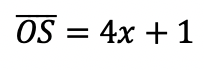median,  to find the value of median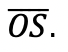Want more practice?  Your wish is my command! Check out the practice problems below:

Practice Questions:

1.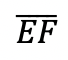is the median of trapezoid ABCDEF, find the value of the median, given the following:2.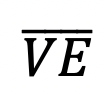is the median of trapezoid ACTIVE, find the value of the median, given the following:3.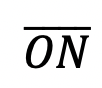is the median of  trapezoid DRAGON, find the value of the median, given the following:

4.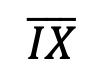is the median of trapezoid MATRIX, find the value of the median, given the following:

Solutions:

Need more of an explanation?  Check out the detailedand practice problems. Happy calculating! 🙂

## Solving Radical Equations: Algebra 2/Trig.

Today we’re back with Algebra 2, this time solving for radical equations!  Did you say “Radical Equations?” As in wild and crazy equations? No, not exactly, radicals in math are used to take the square root, cubed root, or whatever root of a number.

## Example #1:

Radicals are actually pretty cool because we can write them a couple of different ways and they all mean the same thing! Check it out below:Still not sure of their coolness? Let’s see what they look like with actual numbers:
Example: Solve the following algebraic equation below for the missing variable (aka, solve for x).Explanation:

How do I answer this question?

The question wants us to solve for x using our knowledge of radicals and algebra. You can also check out how to solve this question on Youtube here!

How do we do this?

Step 1: We start solving this radical equation like any other algebraic problem: by getting x alone. We can do this easily by subtracting 7 and then dividing out 5.

Step 2: Now, to get rid of that pesky radical, we need to square the entire radical.  Remember, whatever we do to one side of the equation, we must also do to the other side of the equation, therefore, we also square 14 on the other side of the equal sign. *This gets rid of our radical and allows us to solve for x algebraically as normal!

What happens when there is a cubed root though!?!?

When dividing polynomials with different value roots, raise the entire radical to that same power of root to cancel it out:Remember, we know radicals can also be written as fractions:

Therefore we also know that if we raise the entire radical expression to the same power of the root, the two exponents will cancel each other out:

## Example #2:

Want more practice? Try solving radical equations in the next few examples on your own.

## Solutions:

Looking to brush up on how to solve absolute value equations? Check out the post here! Did I miss anything?  Don’t let any questions go unchecked and let me know in the comments! Happy calculating! 🙂

Don’t forget to check out the latest free videos and posts with MathSux and subscribe!

## Earth Day Fractals!

In honor of Earth Day last week, I thought we’d take a look at some math that appears magically in nature.  Math? In nature?  For those of you who think math is unnatural or just terrible in general, this is a great time to be proven otherwise!

The key that links math to nature is all about PATTERNS. All math is based on is patterns.  This includes all types of math, from sequences to finding x, each mathematical procedure follows some type of pattern. Meanwhile back in the nearest forest, patterns are occurring everywhere in nature.

The rock star of all patterns would have to be FRACTALS. A Fractal is a repeating pattern that is ongoing and has different sizes of the exact same thing!  And the amazing thing is that we can actually find fractals in our neighbor’s local garden.

Let’s look at some Fractal Examples:

(1) Romanesco Broccoli:  Check out those repeating shapes, that have the same repeating shapes on those shapes and the same repeating shapes on even smaller shapes and…. my brain hurts!

######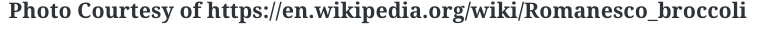(2) Fern Leaves:  The largest part of this fractal is the entire fern leaf itself.  The next smaller and identical part is each individual “leaf” along the stem.  If you look closely, the pattern continues!

######(3) Leaves:  If you’ve ever gotten up real close to any type of leaf, you may have noticed the forever repeating pattern that gets smaller and smaller. Behold the power and fractal pattern of this mighty leaf below!

######.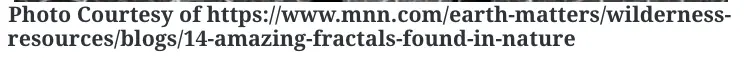Just in case fractals are still a bit hard to grasp, check out the most famous Fractal below,  otherwise known as Sierpinski’s Triangle.  This example might not be found in your local back yard, but it’s the best way to see what a fractal truly is up close and infinite and stuff.

Looking for more math in nature?  Check out this post on the Golden Ratio and happy calculating! 🙂

|    |